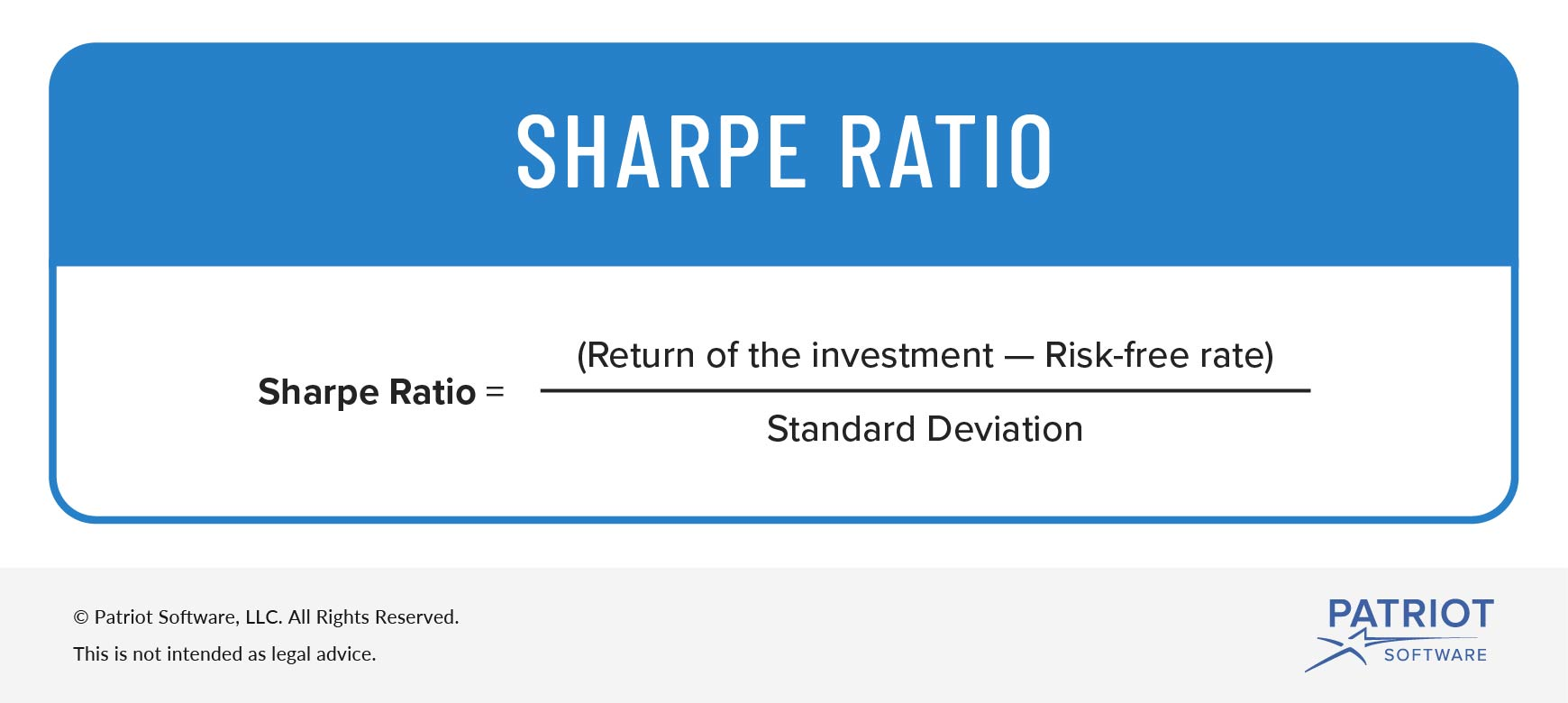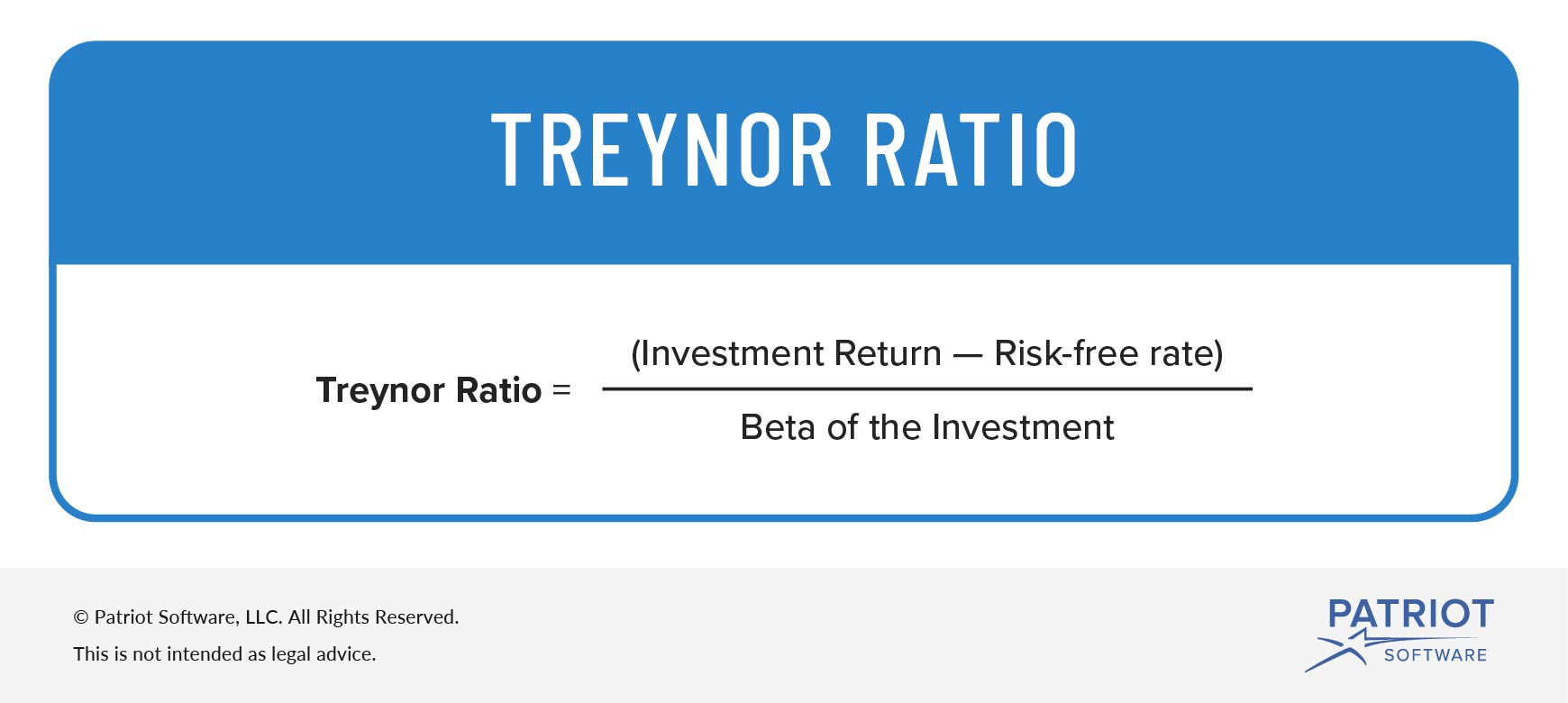Risk-adjusted Return | Definition, Formulas, & More
We are committed to providing timely updates regarding COVID-19.

# What Is a Risk-adjusted Return?Investors compare many risk factors when looking at whether a business is worth investing in. One of the financial aspects investors might look at is your business’s risk-adjusted return. So, what is a risk-adjusted return?

## What is a risk-adjusted return?

A risk-adjusted return is when you or an investor (e.g., small business angel investors) measures the amount of risk involved in an investment’s return. With a risk-adjusted return, you can also compare risk to your potential reward.

Basically, a risk-adjusted return is how much return your investment makes relative to the amount of risk the investment has.

Generally, risk-adjusted returns are represented as numbers or ratings. In most cases, a risk-adjusted return applies to investment funds, individual securities, and portfolios.

Risk-adjusted returns measure certain factors including:

1. Risk management
2. How well the risk performs or pays off

Risk-adjusted returns allow investors to analyze high-risk versus low-risk investments. Typically, the lower the risk, the better the risk-adjusted return.

There are a few ways you can calculate risk-adjusted return by using ratios and formulas. Some calculations can be more complicated than others. While comparing investments, many investors look at multiple risk measures and compare results.

To simplify the process, you can measure risk by using either the Sharpe ratio or the Treynor ratio.

### Sharpe ratio

The Share ratio was developed to help investors understand the return of an investment by comparing it to its risk. It is the most popular risk-measuring method. Essentially, the ratio calculates the average return earned (e.g., similar risks).

To calculate the Sharpe ratio, subtract the risk-free rate from the return of the investment. The risk-free rate is the rate of return of an investment with no risk.

Then, divide that number by the standard deviation of the investment’s excess return. The standard deviation compares an investment’s returns to its average return.

Sharpe ratio = Return of the investment – Risk-free rate / Standard deviation

#### Sharpe ratio example

All of this ratio and formula talk can get a little confusing. So let’s take a look at an example of using the Sharpe ratio.

Say you want to compare two investments, Investment A and B. Investment A returned 20% over the past year and had a standard deviation of 7%. Investment B returned 15% and had a standard deviation of 4%. The risk-free rate was 3% for both investments. The Sharpe ratios for both investments would look like this:

Investment A: (20% – 3%) / 7% = 2.43
Investment B: (15% – 3%) / 4% = 3

Investment A’s risk-adjusted return is 2.43 while Investment B’s is 3. Even though Investment A has a higher return, Investment B has a higher risk-adjusted return.### Treynor ratio

The Treynor ratio, or the reward-to-volatility ratio, helps determine how much return there is for each unit of risk for an investment.

The Treynor ratio is very common to the Sharpe ratio. However, instead of using standard deviation like the Sharpe ratio, the Treynor ratio uses beta.

In the formula, beta measures the change of an investment’s return in response to changes in the overall market.

To calculate the Treynor ratio, use the following formula:

Treynor ratio = Investment return – Risk-free rate / Beta of the investment

#### Treynor ratio example

Let’s take a look at an example of the Treynor ratio. To keep it simple, we will use the same data from the Sharpe ratio example.

As a reminder, Investment A returns 20% and Investment B returns 15%. Both investments have a risk-free rate of 3%. The beta for Investment A and B is 0.50. Plug the information into the Treynor ratio formula to get your totals.

Investment A: (20% – 3%) / 0.50 = 0.34
Investment B: (15% – 3%) / 0.50 = 0.24

Investment A is 0.34 and Investment B is 0.24. As you can see, Investment A has a higher Treynor ratio than Investment B. Because Investment A has a higher Treynor ratio, it is earning more return per unit of risk than Investment B.Need a way to streamline your accounting process and easily track your business’s transactions? Patriot’s online accounting software lets you import bank transactions, create invoices, and more. What are you waiting for? Start your self-guided demo today!

Like what you read? Let’s connect, friend! Like us on Facebook and let’s get talking.